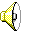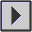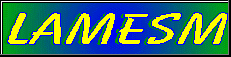## The synthesis of the subsets

We produced a variety of different sounds using the subsets commented in "The definition of the frequency sets and subsets": each frequency set produced 7 sounds. The setup of the other Chaosynth parameters for these sounds were fixed as follows:

r1 = 0.92
r2 = 1.22
k = 4.4
grid = 20 x 20
duration (of the grains) = 0.035 secs
amount of oscillators = 25

The Csound score file generated for the Jup(1) subset, for example, is as follows [ Jup(1) ]

An excerpt of the sound produced by the Jup(1) subset is as follows (WAV, 16 bit mono, 44.1 kHz, 442 KB):#### Comparative listening

By comparing these sounds, we identified the following characteristics:

a) in general, Chaosynth sounds tend to change their "coloration" during their development;

b) all sounds tend to begin with wide distribution of partials and then settle to a periodic fluctuation; sometimes they reach a permanently steady state

c) sounds of subset type 1 are more complex because this subset has more values for the calculation of the spectrum of the particles, whilst sounds of subsets types 4, 5, 6 and 7 are less complex because they have less values for this calculation

d) the sounds of subsets types 2, 4 and 5 are lower in pitch than the sounds of subsets types 3, 6 and 7 because the former subsets contain lower frequencies, whilst the latter contain higher frequencies for the calculation of the spectra of the particles

e) in general, those subsets which have more elements, produce sounds with a richer spectral variation during their development

```BACK to Chaosynth mainNEXT
```Laboratório de Música Eletroacústica de Santa Maria
Brasil